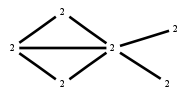# The Markov Bases Database

## G111g_bin

The binary graphical model of G111.

It is a hierarchical model of 6 variables. The dimension of the model is 15.The cardinality of the statespace is 64.

### Properties of the Markov basis

 Markov degree 2 444

 degree 2 444

The model has the following properties:

• All variables are binary.
• It is a graphical model.
• The semigroup is normal.### FILES

 Markov basis: sufficient statistics matrix: G111g_bin.mar (83.69 kb) G111g_bin.mat (3.03 kb) G111g_bin.mod (45 b) G111g_bin.tar.gz (6.94 kb)